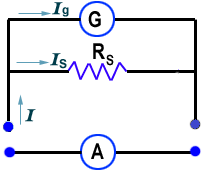# Conversion of Galvanometer into an Ammeter

A Galvanometer is an electrical device which detects the electrical current in a circuit.It also gives the direction of current in a circuit and can measure small amount of current. To convert a galvanometer in an ammeter, we connect a low resistance called shunt across the coil of the galvanometer.Let ‘G’ be the resistance of the coil of the galvanometer and Ig be the amount of current at which the galvanometer gives full scale deflection. Let ‘S’ be the resistant of the shunt connected across the coil of the galvanometer,so that the galvanometer becomes ammeter of range(0-I) A. Now,
$$\text{p.d across ‘S’ = p.d across ‘G’}$$
$$\text{I – I}_g. \text{S} = \text{I}_g.\text{G}$$
$$\text{or, S} = \frac{\text{I}_g}{\text{I}_g\text{I}}.\text{G}$$
$$\frac{1}{\text{R}_\text{net}} = \frac{1}{\text{S}} + \frac{1}{\text{G}}$$

Let  Rnet be the net resistance of the ammeter. Now,
$$\text{or,} \frac{1}{\text{R}_\text{net}} = \frac{\text{G + S}}{\text{G.S}}$$
$$\therefore {\text{R}_\text{net}} = \frac{G.S}{\text{G + S}}$$

So, the resistance of ammeter is less than that of shunt. Since S is low resistance, resistance of ammeter RA is very low and when it is connected in series in the circuits, it will not affect the current passing through the circuit.

Do you like this article ? If yes then like otherwise dislike : 2

#### One Response to “Conversion of Galvanometer into an Ammeter”

1.